# Logarithm Worksheets

Logarithm worksheets for high school students cover the skills based on converting between logarithmic form and exponential form, evaluating logarithmic expressions, finding the value of the variable to make the equation correct, solving logarithmic equations, single logarithm, expanding logarithm using power rule, product rule and quotient rule, expressing the log value in algebraic expression, logarithms using calculator and more. Explore some of these exercises for free!

Logarithmic and Exponential Form

There are two sections in each printable worksheet. The first section is about converting logarithmic form to exponential form. Second section is vice versa.Only Numerals:

Grab 'em All

Numerals & Variables:

Evaluating Expressions: Level 1

Evaluate the value of each logarithmic expression using power rule.Easy:

Grab 'em All

Moderate:

Difficult:

Evaluating Expressions: Level 2

Solve the problems involving more logarithmic expressions. Take enough practice with level 1 and come back here.Easy:

Moderate:

Difficult:

Solving for x: Level 1

Rewrite each logarithmic equation in exponential form and solve for the variable in these pdf worksheets.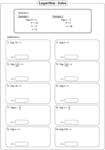Easy:

Grab 'em All

Moderate:

Grab 'em All

Solving for x: Level 2

Find the unknown variable that makes the logarithmic equation sentence correct.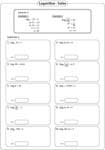Easy:

Moderate:

Grab 'em All

Solving Logarithmic Equations

Solve the logarithmic equations and find the value of the unknown variables. On easy and moderate-level printables, you will end up with linear equations after canceling out the logarithms. Difficult level contains quadratic equations.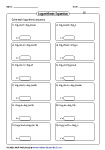Easy:

Moderate:

Grab 'em All

Difficult:

Single Logarithm and Expansion

Applying the logarithm rules, such as product rule, quotient rule and power rule, rewrite each expression in single logarithm or expanded form.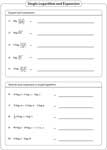Express in Algebraic Expression

Each variable in this set of high school worksheet pdfs is assigned with a logarithm. Translate each logarithmic expression into algebraic expression.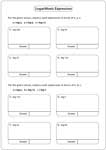Logarithm Value using Calculator

Use calculator to find the value of each logarithm. Round the answer to two decimal places. Mixed type contains a both common and natural logarithm.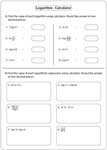Common Logarithm:

Natural Logarithm:

Mixed Logarithm:

Change of Base Rule

Rewrite each logarithm by applying the change of base rule; find the value using the calculator. Round the answer to two decimal places.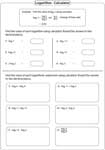Solving Equations using Calculator

Using either antilogarithm method or exponential form method, solve each logarithmic equation.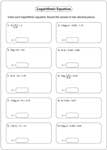Common Logarithm:

Natural Logarithm:

Mixed Logarithm:

Related Worksheets